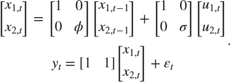# Create State-Space Model with Random State Coefficient

This example shows how to create a time-varying, state-space model containing a random, state coefficient.

Write a function that specifies how the parameters in params map to the state-space model matrices, the initial state values, and the type of state. Symbolically, the model isis a random coefficient.

 % Copyright 2015 The MathWorks, Inc. function [A,B,C,D] = randomCoeffParamMap(c) % State-space model parameter-to-matrix mapping function with a random % coefficient example. There are two states: one is a random walk with % disturbance variance 1, and the other is a first-order Markov model with % a random coefficient and an unknown variance. The observation equation % is the sum of the two states, and the innovation has variance 1. A = diag([1,c(1)*rand]); B = [1 0; 0 c(2)]; C = [1,1]; D = 1; end 

Create the state-space model by passing randomCoeffParamMap as a function handle to ssm.

rng('default'); % For reproducibility Mdl = ssm(@randomCoeffParamMap); 

ssm implicitly creates the ssm model Mdl.

Display Mdl using disp. Specify initial parameters values.

disp(Mdl,[3; 5]) 
State-space model type: <a href="matlab: doc ssm">ssm</a> State vector length: 2 Observation vector length: 1 State disturbance vector length: 2 Observation innovation vector length: 1 Sample size supported by model: Unlimited State variables: x1, x2,... State disturbances: u1, u2,... Observation series: y1, y2,... Observation innovations: e1, e2,... State equations: x1(t) = x1(t-1) + u1(t) x2(t) = (0.38)x2(t-1) + (5)u2(t) Observation equation: y1(t) = x1(t) + x2(t) + e1(t) Initial state distribution: Initial state means x1 x2 0 0 Initial state covariance matrix x1 x2 x1 1e+07 0 x2 0 1e+07 State types x1 x2 Diffuse Diffuse 

disp sets the parameters to their initial values, or functions of their initial values. In this case, the first parameter is the initial values times a random number.